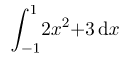## Welcome to the World of Modelling and Simulation

### What is Modelling?

This blog is all about system dynamics modelling and simulation applied in the engineering field, especially mechanical, electrical, and ...### Implicit Euler Method by MATLAB to Solve an ODE

In this example, an implementation of the Implicit Euler approach by MATLAB program to solve an ordinary differential equation (ODE) is presented. Let's consider a differential equation, which is defined as,

dv/dt = p(t) v + q(t)

Where,

p(t) = 5(1+t)

and,

q(t) = (1+t)e-t

The initial value is, v(0) = 1; and the time period is 0 < t < 10.

Implicit Euler approach is unconditionally stable. The implementation of Implicit Euler scheme may be represented as,

v_n+1 = (v_n + hq_n+1) / (1 + hp_n+1)

The following MATLAB program implements this scheme:

MATLAB program:

close all;
clc;
y1(1) = 0; % Initial condition
h = 0.1; % Time step
t1(1) = 0;
i = 1;

% Implementation of the Implicit Euler approach
while (t1(i) <= 30)
q=(1+t1(i))*exp(-t1(i));
p= 5*(1+t1(i));

y1(i+1) = (y1(i)+(h*q))/(1+h*p);
t1(i+1) = t1(i) + h;
i = i + 1;
end
plot(t1,y1,'-o')
hold on

Program Output:
The following plot shows the progression of the solution as the time increases.

### Explicit Euler Method by MATLAB to Solve an ODE

In this example, an implementation of the Explicit Euler approach by MATLAB program to solve an ordinary differential equation (ODE) is presented. Let's consider a differential equation, which is defined as,

dv/dt = p(t) v + q(t)

Where,

p(t) = 5(1+t)

and,

q(t) = (1+t)e^-t

The initial value is, v(0) = 1;, and the time period is 0 < t < 10.

The implementation of Explicit Euler scheme may be represented as,

v_n+1 = v_n (1 - ph) + hq

The following MATLAB program implements this scheme:

close all;
clc;
y_initial = 1; % Defines initial condition, v(0)=1
h = 1.1; % Defines time step
[ t, v ] = expliciteuler( @f_ode, [ 0.0,10 ], y_initial, h );
plot(t,v,'--*')
hold on

function f_vt = f_ode ( t, v )
% This function defines the differential equation
% t is the independent variable
% v is the dependent variable
% f_vt represents the dv/dt
p= 5*(1+t);
q=(1+t)*exp(-t);
f_vt = p*v+q;

function [x, y] = expliciteuler( f_ode, xRange, y_initial, h )
% This function uses Euler’s explicit method to solve the ODE
% dv/dt=f_ode(t,v); x refers to t and y refers to v
% f_ode defines the differential equation of the problem
% xRange = [x1, x2] where the solution is sought on
% y_initial = column vector of initial values for y at x1
% numSteps = number of equally-sized steps to take from x1 to x2
% x = row vector of values of x
% y = matrix whose k-th column is the approximate solution at x(k)
x(1) = xRange(1);
numSteps = ( xRange(2) - xRange(1) ) /h ;
y(:,1) = y_initial;
for k = 1 : numSteps
x(1,k+1) = x(1,k) + h;
y(:,k+1) = y(:,k) + h * f_ode( x(k), y(:,k) );

end

Program Output:

The following plot shows the value of the solution with respect of time increment.

### Learning Mathematica, Lesson 3: Integration

Integration becomes very handy if you choose to use Wolfram Mathematica. You can evaluate definite and indefinite integrals using two ways.

1. You may use "Integrate[f, x]" command for the indefinite integrals, and "Integrate[f, {x, upper limit, lower limit}]" for the definite integrals.

2. Useintto enter  and then useto enter the lower limit, thenfor the upper limit:

For example, if you would like to find out the following integral of a function using both approaches,

Now, let's look at another example, where you will find detailed Mathematica codes to determine the integral of the following:Note that the following Mathematica program also implements a numerical integration scheme by using a built-in "NIntregate" command. Look carefully the systaxes used in the following codes to display the results, you may represent them in your own fashion.

Mathematica Codes:
(*Showing Exact Integration of First Problem*)
Print["Exact solution of \!\(\*SubsuperscriptBox[\(\[Integral]\), \
\(-1\), \(1\)]\)(2\!\(\*SuperscriptBox[\(x\), \(2\)]\)+3)\
\[DifferentialD]x"]
FullSimplify[\!\(
\*SubsuperscriptBox[\(\[Integral]\), \(-1\), \(1\)]\(\((2
\*SuperscriptBox[\(x\), \(2\)] + 3)\) \[DifferentialD]x\)\)]

(*Showing Numerical Integration of First Problem*)
Print["Numerical Integration by Approximation: \
\!\(\*SubsuperscriptBox[\(\[Integral]\), \(-1\), \
\(1\)]\)(2\!\(\*SuperscriptBox[\(x\), \(2\)]\)+3)\[DifferentialD]x"]
NIntegrate[(2 x^2 + 3), {x, -1, 1}]
Print["Numerical Integration by Gaussian Rule Order-1: \
\!\(\*SubsuperscriptBox[\(\[Integral]\), \(-1\), \
\(1\)]\)(2\!\(\*SuperscriptBox[\(x\), \(2\)]\)+3)\[DifferentialD]x"]
a = 2 x^2 + 3 /. x -> -Sqrt[1/(3)];
b = 2 x^2 + 3 /. x -> Sqrt[1/(3)];

c = N[a + b]

Program Output:

### A Mathematica Program for the Newton Raphson Method

The following Mathematica program implements the widely used Newton Raphson approach. Any functions may be considered here, and the following program is ready to evaluate it.

(*Mathematica Codes for Newton-Raphson Approach*)
Sigma = 100000*(Sqrt[epsilon] + 1/80*Sin[Pi*epsilon/0.002]) - 4000;
epsilontable = {0.0002};
Error = {1};
SetTolerance = 0.0005;
MaximumIteration = 100;
i = 1;

(*Newton-Raphson Algorithm Implementation*)
While[And[i <= MaximumIteration, Abs[Error[[i]]] > SetTolerance],
epsilonnew =
epsilontable[[i]] - (Sigma /.
epsilon -> epsilontable[[i]]);
epsilontable = Append[epsilontable, epsilonnew];
Errornew = (epsilonnew - epsilontable[[i]])/epsilonnew;
Error = Append[Error, Errornew];
i++];

L = Length[epsilontable];
SolutionTable =
Table[{i - 1, epsilontable[[i]], Error[[i]]}, {i, 1, L}];
SolutionTable1 = {"Iteration Number", "Epsilon", "Error"};
L = Prepend[SolutionTable, SolutionTable1];
Print["Showing Results for the Newton-Raphson Approach when Sigma is \
4,000 and Initial Guess is 0.0002"]
ScientificForm[L // MatrixForm, 4]

Program Output: